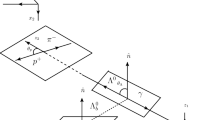# $${\Lambda}_c^{+}$$ polarimetry using the dominant hadronic mode

## Abstract

The polarimeter vector field for multibody decays of a spin-half baryon is introduced as a generalisation of the baryon asymmetry parameters. Using a recent amplitude analysis of the $${\Lambda}_c^{+}$$pKπ+ decay performed at the LHCb experiment, we compute the distribution of the kinematic-dependent polarimeter vector for this process in the space of Mandelstam variables to express the polarised decay rate in a model-agnostic form. The obtained representation can facilitate polarisation measurements of the $${\Lambda}_c^{+}$$ baryon and eases inclusion of the $${\Lambda}_c^{+}$$ → pKπ+ decay mode in hadronic amplitude analyses.

### Heavy baryons as polarimeters at colliders

Mario Galanti, Andrea Giammanco, … Jure Zupan### Radiative b-baryon decays to measure the photon and b-baryon polarization

Luis Miguel García Martín, Brij Jashal, … Rahul Sinha